# Specific requirements

In this programming project, complete the following steps:
• Select a data set and load it into Python/R.
• Perform kernelized SVMs on the data of your own choosing.
• Also perform other 2 classification models on the same data.
• Compare and contrast the results of kernelized SVMs and the 2 classification models.
• Submit a *.html file generated by Jupyter Notebook/R Markdown.

# Data frame information

### Description

Dataset of Social media ads describing users, whether users have purchased a product by clicking on the advertisements shown to them.

### Content

Social media ads of 400 rows and 5 columns.

#### User ID

Character field which catpures the user account number.

#### Gender

Character field which indicates whether the user is female or male.

#### Age

The age of the user.

#### Estimated Salary

The estimated salary of the user.

#### Purchased

A binary field which indicates whether the user made the purchase.

# Clearing R Studio Memory Usage

``gc()``
``````##           used (Mb) gc trigger (Mb) max used (Mb)
## Ncells  543378 29.1    1241842 66.4   621331 33.2
## Vcells 1026080  7.9    8388608 64.0  1600889 12.3``````
``rm(list = ls())``

# Time Counter Start

``start_time <- Sys.time()``

# Include the knitr package for integration of R code into Markdown

``knitr::opts_chunk\$set(echo = TRUE)``

# All the libraries used in this code

``````library(easypackages)

# Import data

``````oldw <- getOption("warn")
options(warn = -1)
col_types = cols(
Age = col_number(),
EstimatedSalary = col_number(),
Gender = col_character(),
Purchased = col_character(),
`User ID` = col_character()
)
)
options(warn = -1) ``````

# Numeric/character field separator

``````num.names <- input_data %>% select_if(is.numeric) %>% colnames()
ch.names <- input_data %>% select_if(is.character) %>% colnames()``````

# Descriptive statistics before data processing

### Dimension of data frame

``dim(input_data)``
``##  400   5``

### Structure of data frame

``str(input_data)``
``````## tibble [400 x 5] (S3: spec_tbl_df/tbl_df/tbl/data.frame)
##  \$ User ID        : chr [1:400] "15624510" "15810944" "15668575" "15603246" ...
##  \$ Gender         : chr [1:400] "Male" "Male" "Female" "Female" ...
##  \$ Age            : num [1:400] 19 35 26 27 19 27 27 32 25 35 ...
##  \$ EstimatedSalary: num [1:400] 19000 20000 43000 57000 76000 58000 84000 150000 33000 65000 ...
##  \$ Purchased      : chr [1:400] "0" "0" "0" "0" ...
##  - attr(*, "spec")=
##   .. cols(
##   ..   `User ID` = col_character(),
##   ..   Gender = col_character(),
##   ..   Age = col_number(),
##   ..   EstimatedSalary = col_number(),
##   ..   Purchased = col_character()
##   .. )``````

### Summary statistics of data frame

``summary(input_data)``
``````##    User ID             Gender               Age        EstimatedSalary
##  Length:400         Length:400         Min.   :18.00   Min.   : 15000
##  Class :character   Class :character   1st Qu.:29.75   1st Qu.: 43000
##  Mode  :character   Mode  :character   Median :37.00   Median : 70000
##                                        Mean   :37.66   Mean   : 69743
##                                        3rd Qu.:46.00   3rd Qu.: 88000
##                                        Max.   :60.00   Max.   :150000
##   Purchased
##  Length:400
##  Class :character
##  Mode  :character
##
##
## ``````

### Glimpse of data frame

``glimpse(input_data)``
``````## Rows: 400
## Columns: 5
## \$ `User ID`       <chr> "15624510", "15810944", "15668575", "15603246", "15...
## \$ Gender          <chr> "Male", "Male", "Female", "Female", "Male", "Male",...
## \$ Age             <dbl> 19, 35, 26, 27, 19, 27, 27, 32, 25, 35, 26, 26, 20,...
## \$ EstimatedSalary <dbl> 19000, 20000, 43000, 57000, 76000, 58000, 84000, 15...
## \$ Purchased       <chr> "0", "0", "0", "0", "0", "0", "0", "1", "0", "0", "...``````

``head(input_data)``
``````## # A tibble: 6 x 5
##   `User ID` Gender   Age EstimatedSalary Purchased
##   <chr>     <chr>  <dbl>           <dbl> <chr>
## 1 15624510  Male      19           19000 0
## 2 15810944  Male      35           20000 0
## 3 15668575  Female    26           43000 0
## 4 15603246  Female    27           57000 0
## 5 15804002  Male      19           76000 0
## 6 15728773  Male      27           58000 0``````

### Tail of data frame

``tail(input_data)``
``````## # A tibble: 6 x 5
##   `User ID` Gender   Age EstimatedSalary Purchased
##   <chr>     <chr>  <dbl>           <dbl> <chr>
## 1 15757632  Female    39           59000 0
## 2 15691863  Female    46           41000 1
## 3 15706071  Male      51           23000 1
## 4 15654296  Female    50           20000 1
## 5 15755018  Male      36           33000 0
## 6 15594041  Female    49           36000 1``````

### Variable data types

``sapply(input_data,mode)``
``````##         User ID          Gender             Age EstimatedSalary       Purchased
##     "character"     "character"       "numeric"       "numeric"     "character"``````

### Mean of each variable

``lapply(input_data[,num.names],mean)``
``````## \$Age
##  37.655
##
## \$EstimatedSalary
##  69742.5``````

### Median of each variable

``lapply(input_data[,num.names],median)``
``````## \$Age
##  37
##
## \$EstimatedSalary
##  70000``````

### Mode of each variable

``lapply(input_data[,num.names],mfv)``
``````## \$Age
##  35
##
## \$EstimatedSalary
##  72000``````

### Minimum value of each variable

``lapply(input_data[,num.names],min)``
``````## \$Age
##  18
##
## \$EstimatedSalary
##  15000``````

### Maximum value of each variable

``lapply(input_data[,num.names],max)``
``````## \$Age
##  60
##
## \$EstimatedSalary
##  150000``````

### Range of each variable

``lapply(input_data[,num.names],range)``
``````## \$Age
##  18 60
##
## \$EstimatedSalary
##   15000 150000``````

### Variance of each variable

``lapply(input_data[,num.names],var)``
``````## \$Age
##  109.8907
##
## \$EstimatedSalary
##  1162602701``````

### Standard deviation of each variable

``lapply(input_data[,num.names],sd)``
``````## \$Age
##  10.48288
##
## \$EstimatedSalary
##  34096.96``````

### Median absolute deviation of each variable

``lapply(input_data[,num.names],mad)``
``````## \$Age
##  11.8608
##
## \$EstimatedSalary
##  31134.6``````

# Data Processing

## Converting character variables into factors

### Explanation

To ensure that R’s data science models work correctly, all categorical dependent variables must be explicitly converted into factors. As for the independent variables, if the variable is both categorical and has more than two levels, then it should be converted into a factor.

``````input_data <- as.data.frame(lapply(input_data, function(x) if(is.character(x)){
x=as.factor(x)
} else x))``````

## Sorting data set

### Explanation

Useful for examinating the data values. By sorting the data, one can tell if there are missing or corrupted data values.

``````input_data <- input_data[order(input_data[,1]),]
glimpse(input_data)``````
``````## Rows: 400
## Columns: 5
## \$ User.ID         <fct> 15566689, 15569641, 15570769, 15570932, 15571059, 1...
## \$ Gender          <fct> Female, Female, Female, Male, Female, Female, Male,...
## \$ Age             <dbl> 35, 58, 26, 34, 33, 21, 40, 35, 58, 35, 48, 35, 41,...
## \$ EstimatedSalary <dbl> 57000, 95000, 80000, 115000, 41000, 16000, 71000, 5...
## \$ Purchased       <fct> 0, 1, 0, 0, 0, 0, 1, 0, 1, 0, 1, 0, 0, 0, 1, 1, 0, ...``````
``````input_data <- input_data[order(-input_data[,1]),]
glimpse(input_data)``````
``````## Rows: 400
## Columns: 5
## \$ User.ID         <fct> 15566689, 15569641, 15570769, 15570932, 15571059, 1...
## \$ Gender          <fct> Female, Female, Female, Male, Female, Female, Male,...
## \$ Age             <dbl> 35, 58, 26, 34, 33, 21, 40, 35, 58, 35, 48, 35, 41,...
## \$ EstimatedSalary <dbl> 57000, 95000, 80000, 115000, 41000, 16000, 71000, 5...
## \$ Purchased       <fct> 0, 1, 0, 0, 0, 0, 1, 0, 1, 0, 1, 0, 0, 0, 1, 1, 0, ...``````

## Replacing missing values with mean or NA

### Explanation

These missing values could cause inaccuracies or errors when calculating data limits, central tendency, dispersion tendency, correlation, multicollinearity, p-values, z-scores, variance inflation factors, etc.

``````input_data <- as.data.frame(lapply(input_data, function(x) if(is.numeric(x) && is.na(x)){
mean(x, na.rm = TRUE)
} else { if(is.character(x) && is.na(x)){x = "NA"} else x }
))
glimpse(input_data)``````
``````## Rows: 400
## Columns: 5
## \$ User.ID         <fct> 15566689, 15569641, 15570769, 15570932, 15571059, 1...
## \$ Gender          <fct> Female, Female, Female, Male, Female, Female, Male,...
## \$ Age             <dbl> 35, 58, 26, 34, 33, 21, 40, 35, 58, 35, 48, 35, 41,...
## \$ EstimatedSalary <dbl> 57000, 95000, 80000, 115000, 41000, 16000, 71000, 5...
## \$ Purchased       <fct> 0, 1, 0, 0, 0, 0, 1, 0, 1, 0, 1, 0, 0, 0, 1, 1, 0, ...``````

## Scaling numeric variables

### Explanation

These numeric variables are centered and standardized between -1 and 1. In order to correctly calculate the distances between data points, the values of each variable have to be on the same scale. Also, it is easier to fit smaller numbers onto the axes of a graph.

#### Function for standardizing data values

Standardization = (x - mean(x))/std(x)

``````input_data <- as.data.frame(lapply(input_data, function(x) if(is.numeric(x)){
(x - mean(x)) / sd(x)
} else x))

str(input_data)``````
``````## 'data.frame':    400 obs. of  5 variables:
##  \$ User.ID        : Factor w/ 400 levels "15566689","15569641",..: 1 2 3 4 5 6 7 8 9 10 ...
##  \$ Gender         : Factor w/ 2 levels "Female","Male": 1 1 1 2 1 1 2 2 1 1 ...
##  \$ Age            : num  -0.253 1.941 -1.112 -0.349 -0.444 ...
##  \$ EstimatedSalary: num  -0.374 0.741 0.301 1.327 -0.843 ...
##  \$ Purchased      : Factor w/ 2 levels "0","1": 1 2 1 1 1 1 2 1 2 1 ...``````
``glimpse(input_data)``
``````## Rows: 400
## Columns: 5
## \$ User.ID         <fct> 15566689, 15569641, 15570769, 15570932, 15571059, 1...
## \$ Gender          <fct> Female, Female, Female, Male, Female, Female, Male,...
## \$ Age             <dbl> -0.25327018, 1.94078408, -1.11181315, -0.34866384, ...
## \$ EstimatedSalary <dbl> -0.37371367, 0.74075518, 0.30083327, 1.32731773, -0...
## \$ Purchased       <fct> 0, 1, 0, 0, 0, 0, 1, 0, 1, 0, 1, 0, 0, 0, 1, 1, 0, ...``````

# Descriptive statistics after data processing

### Dimension of data frame

``dim(input_data)``
``##  400   5``

### Structure of data frame

``str(input_data)``
``````## 'data.frame':    400 obs. of  5 variables:
##  \$ User.ID        : Factor w/ 400 levels "15566689","15569641",..: 1 2 3 4 5 6 7 8 9 10 ...
##  \$ Gender         : Factor w/ 2 levels "Female","Male": 1 1 1 2 1 1 2 2 1 1 ...
##  \$ Age            : num  -0.253 1.941 -1.112 -0.349 -0.444 ...
##  \$ EstimatedSalary: num  -0.374 0.741 0.301 1.327 -0.843 ...
##  \$ Purchased      : Factor w/ 2 levels "0","1": 1 2 1 1 1 1 2 1 2 1 ...``````

### Summary statistics of data frame

``summary(input_data)``
``````##      User.ID       Gender         Age           EstimatedSalary     Purchased
##  15566689:  1   Female:204   Min.   :-1.87496   Min.   :-1.605495   0:257
##  15569641:  1   Male  :196   1st Qu.:-0.75409   1st Qu.:-0.784308   1:143
##  15570769:  1                Median :-0.06248   Median : 0.007552
##  15570932:  1                Mean   : 0.00000   Mean   : 0.000000
##  15571059:  1                3rd Qu.: 0.79606   3rd Qu.: 0.535458
##  15573452:  1                Max.   : 2.13157   Max.   : 2.353802
##  (Other) :394``````

### Glimpse of data frame

``glimpse(input_data)``
``````## Rows: 400
## Columns: 5
## \$ User.ID         <fct> 15566689, 15569641, 15570769, 15570932, 15571059, 1...
## \$ Gender          <fct> Female, Female, Female, Male, Female, Female, Male,...
## \$ Age             <dbl> -0.25327018, 1.94078408, -1.11181315, -0.34866384, ...
## \$ EstimatedSalary <dbl> -0.37371367, 0.74075518, 0.30083327, 1.32731773, -0...
## \$ Purchased       <fct> 0, 1, 0, 0, 0, 0, 1, 0, 1, 0, 1, 0, 0, 0, 1, 1, 0, ...``````

``head(input_data)``
``````##    User.ID Gender        Age EstimatedSalary Purchased
## 1 15566689 Female -0.2532702      -0.3737137         0
## 2 15569641 Female  1.9407841       0.7407552         1
## 3 15570769 Female -1.1118131       0.3008333         0
## 4 15570932   Male -0.3486638       1.3273177         0
## 5 15571059 Female -0.4440575      -0.8429637         0
## 6 15573452 Female -1.5887815      -1.5761669         0``````

### Tail of data frame

``tail(input_data)``
``````##      User.ID Gender        Age EstimatedSalary Purchased
## 395 15811613 Female -0.1578765       0.1541926         0
## 396 15813113   Male  0.2236981       1.0926927         1
## 397 15814004   Male -1.0164195      -1.4588544         0
## 398 15814553   Male  1.8453904      -0.2857293         1
## 399 15814816   Male -0.6348448      -0.1097605         0
## 400 15815236 Female  0.7006665       1.7965678         1``````

### Variable data types

``sapply(input_data,mode)``
``````##         User.ID          Gender             Age EstimatedSalary       Purchased
##       "numeric"       "numeric"       "numeric"       "numeric"       "numeric"``````

### Mean of each variable

``lapply(input_data[,num.names],mean)``
``````## \$Age
##  -1.050681e-16
##
## \$EstimatedSalary
##  6.539365e-18``````

### Median of each variable

``lapply(input_data[,num.names],median)``
``````## \$Age
##  -0.06248285
##
## \$EstimatedSalary
##  0.007551993``````

### Mode of each variable

``lapply(input_data[,num.names],mfv)``
``````## \$Age
##  -0.2532702
##
## \$EstimatedSalary
##  0.06620825``````

### Minimum value of each variable

``lapply(input_data[,num.names],min)``
``````## \$Age
##  -1.874962
##
## \$EstimatedSalary
##  -1.605495``````

### Maximum value of each variable

``lapply(input_data[,num.names],max)``
``````## \$Age
##  2.131571
##
## \$EstimatedSalary
##  2.353802``````

### Range of each variable

``lapply(input_data[,num.names],range)``
``````## \$Age
##  -1.874962  2.131571
##
## \$EstimatedSalary
##  -1.605495  2.353802``````

### Variance of each variable

``lapply(input_data[,num.names],var)``
``````## \$Age
##  1
##
## \$EstimatedSalary
##  1``````

### Standard deviation of each variable

``lapply(input_data[,num.names],sd)``
``````## \$Age
##  1
##
## \$EstimatedSalary
##  1``````

### Median absolute deviation of each variable

``lapply(input_data[,num.names],mad)``
``````## \$Age
##  1.131445
##
## \$EstimatedSalary
##  0.9131195``````

# Plots and Graphs

## Box plots

### Explanation

This box plot reveals the mean value, minimum value, and maximum value of each variable.

### Observation

It appears that both Age and Estimated Salary are pretty much centered at 0. However, if it is broken down by the factor level of the target variable Purchased, each predictor variable appears to have a higher mean for transactions in which the customers did make a purchase.

``````oldw <- getOption("warn")
options(warn = -1)
boxplot(input_data[,num.names])``````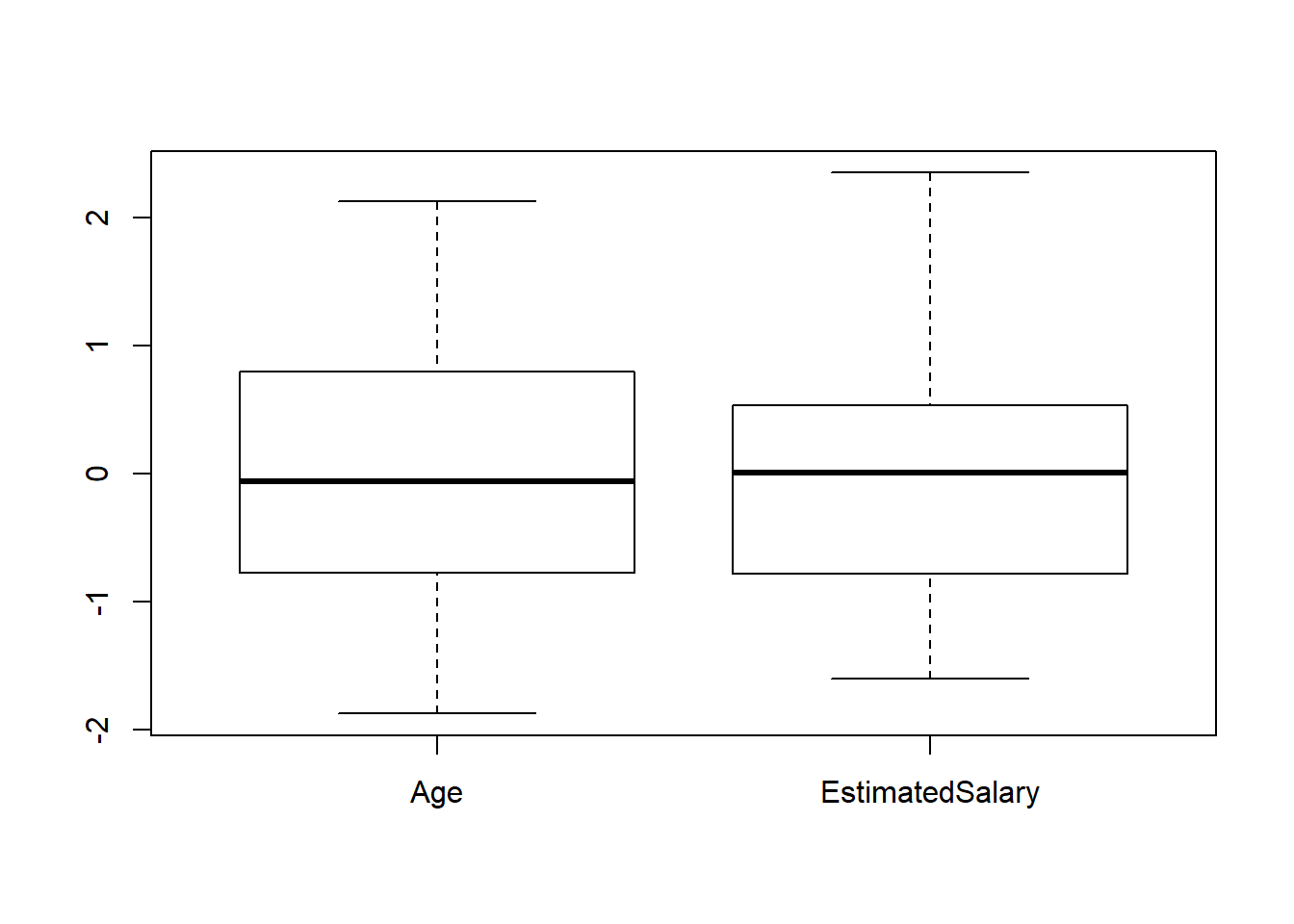``options(warn = oldw)``
``````oldw <- getOption("warn")
options(warn = -1)
ggplot(data = input_data, aes(y=Age)) + geom_boxplot(aes(fill=Purchased))+ggtitle("Box Plot of Age")``````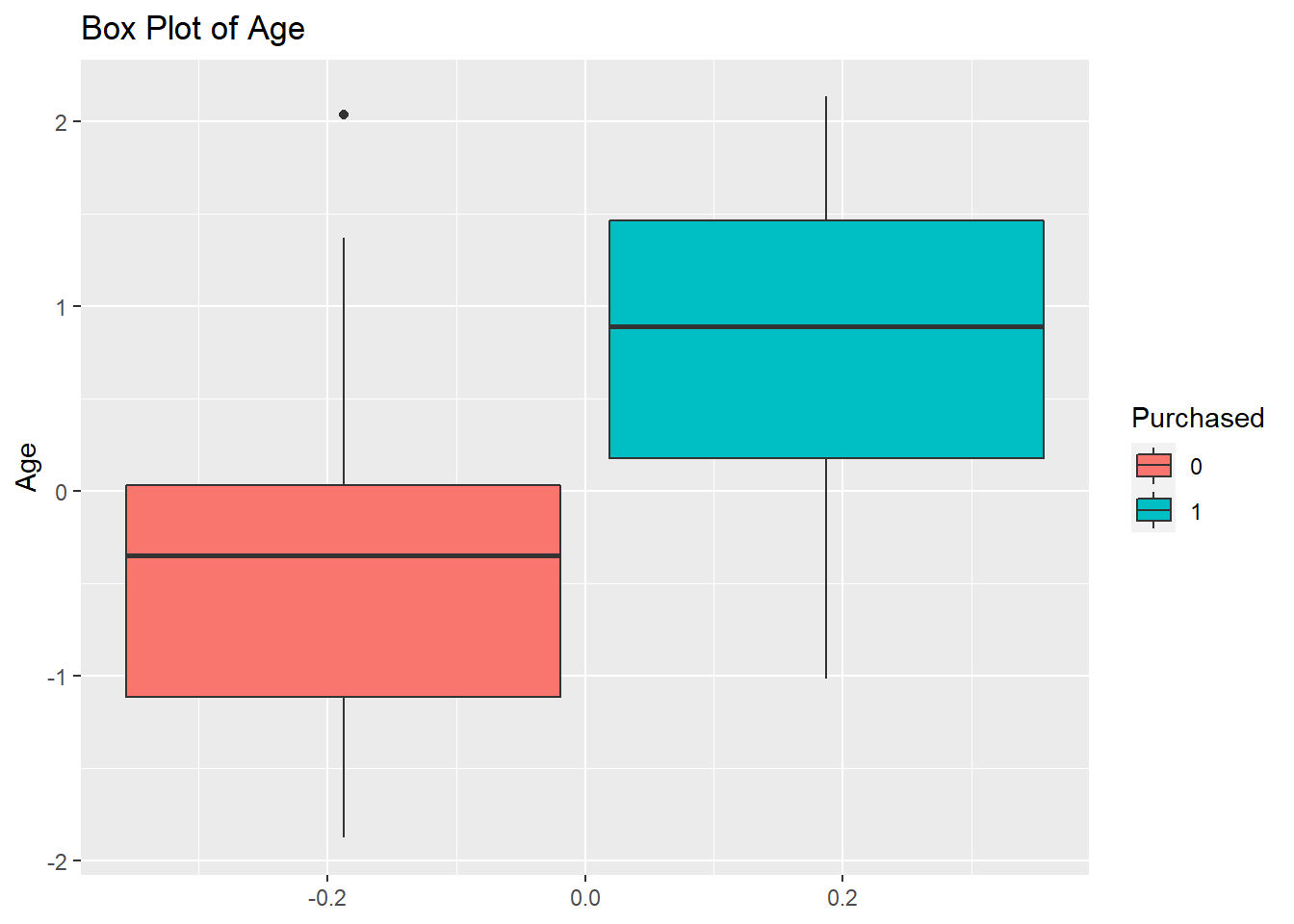``ggplot(data = input_data, aes(y=EstimatedSalary)) + geom_boxplot(aes(fill=Purchased))+ggtitle("Box Plot of Estimated Salary")``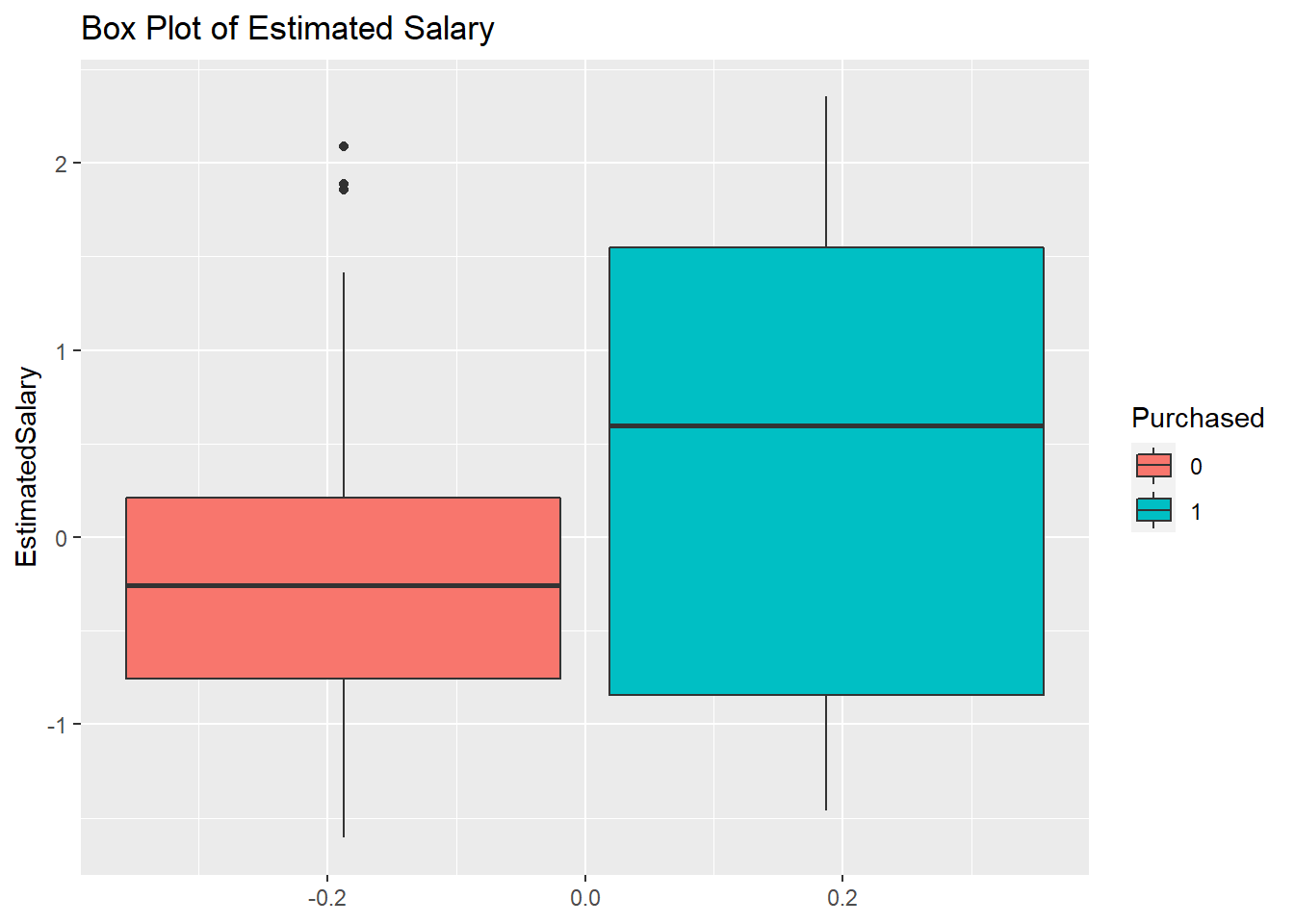``options(warn = oldw)``

## Histograms

### Explanation

The purpose of these histograms is to see the frequency of each predictor variable under each factor level of the target variable.

### Observation

It appears that the majority of the purchases were made by customers who have low salaries.

``````oldw <- getOption("warn")
options(warn = -1)
hist(input_data\$Age, main = "Histogram of Age", xlab="Age")``````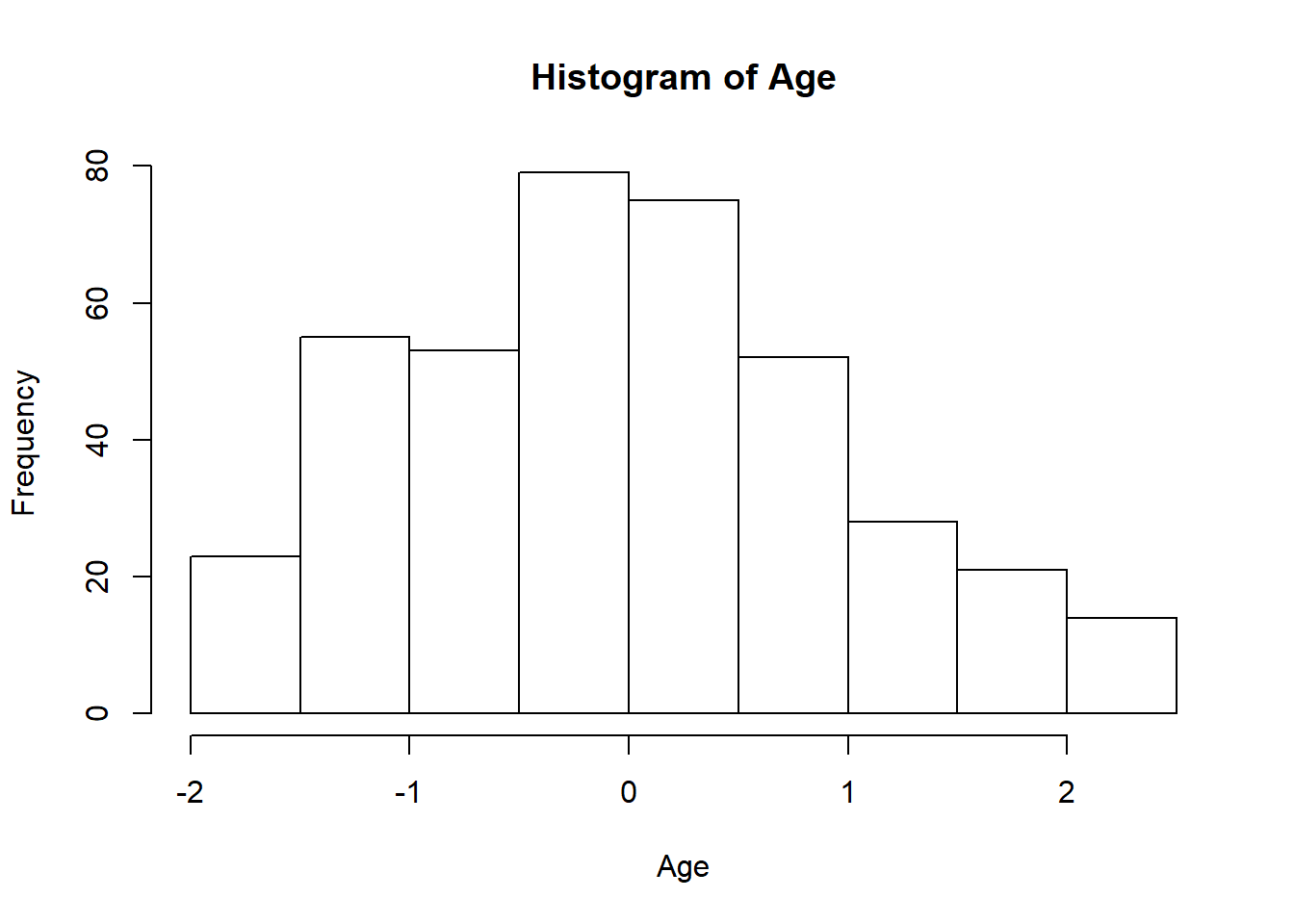``hist(input_data\$EstimatedSalary, main = "Histogram of Estimated Salary", xlab="Estimated Salary")``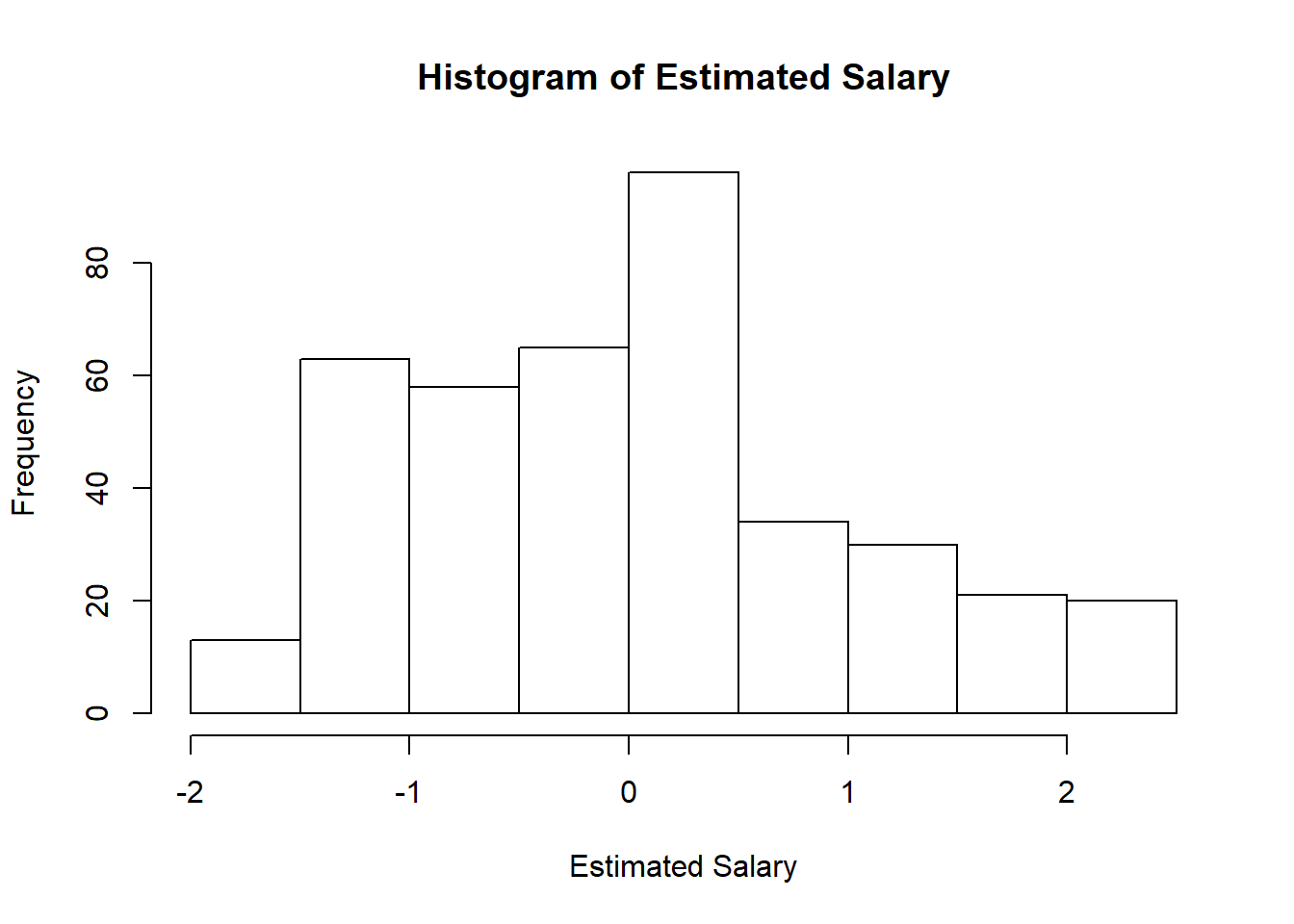``options(warn = oldw)``
``````oldw <- getOption("warn")
options(warn = -1)
ggplot(data = input_data, aes(x=Age, fill=Purchased, color=Purchased)) + geom_histogram(alpha=0.6)+ggtitle("Histogram of Age")``````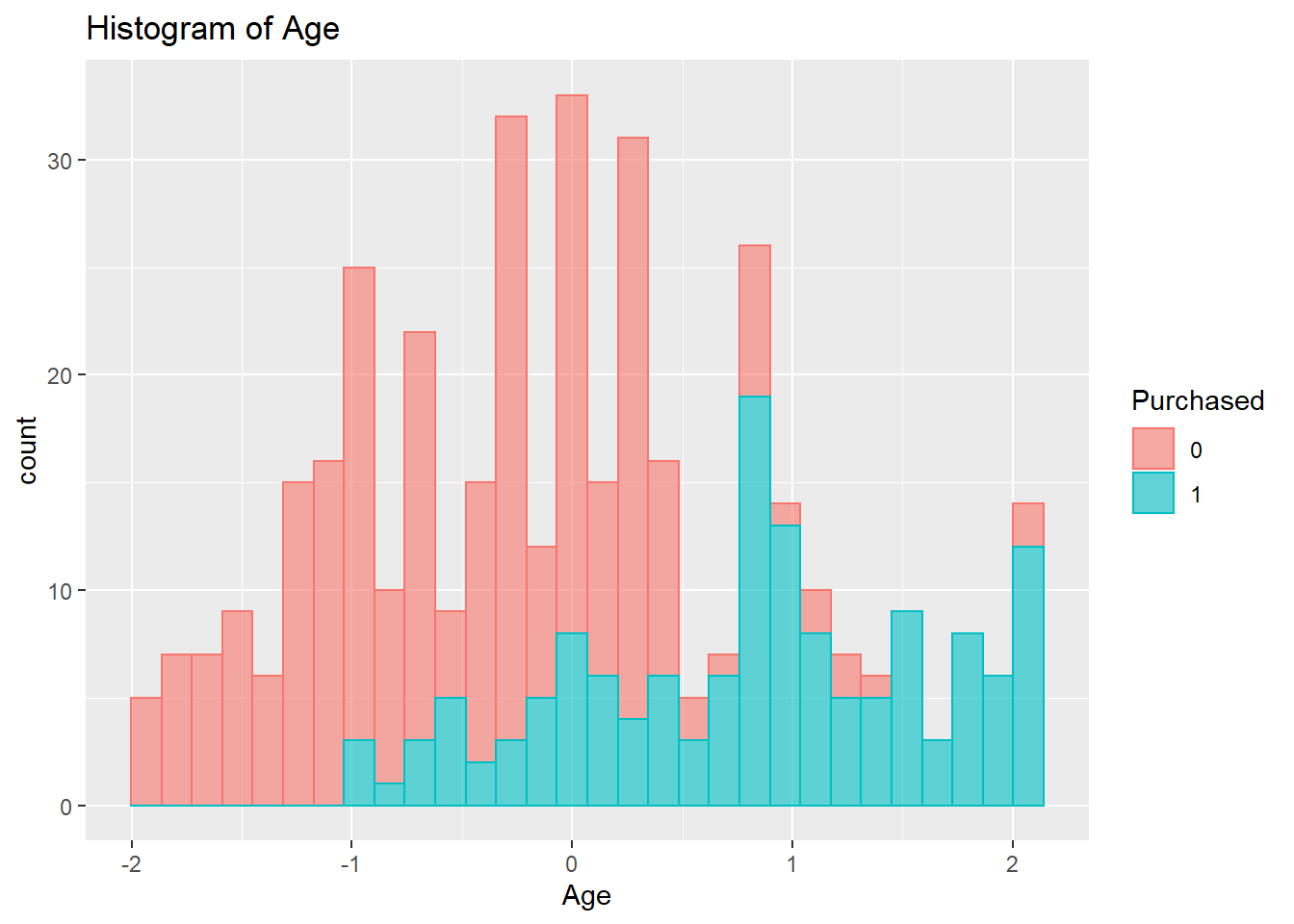``ggplot(data = input_data, aes(x=EstimatedSalary, fill=Purchased, color=Purchased)) + geom_histogram(alpha=0.6)+ggtitle("Histogram of Estimated Salary")``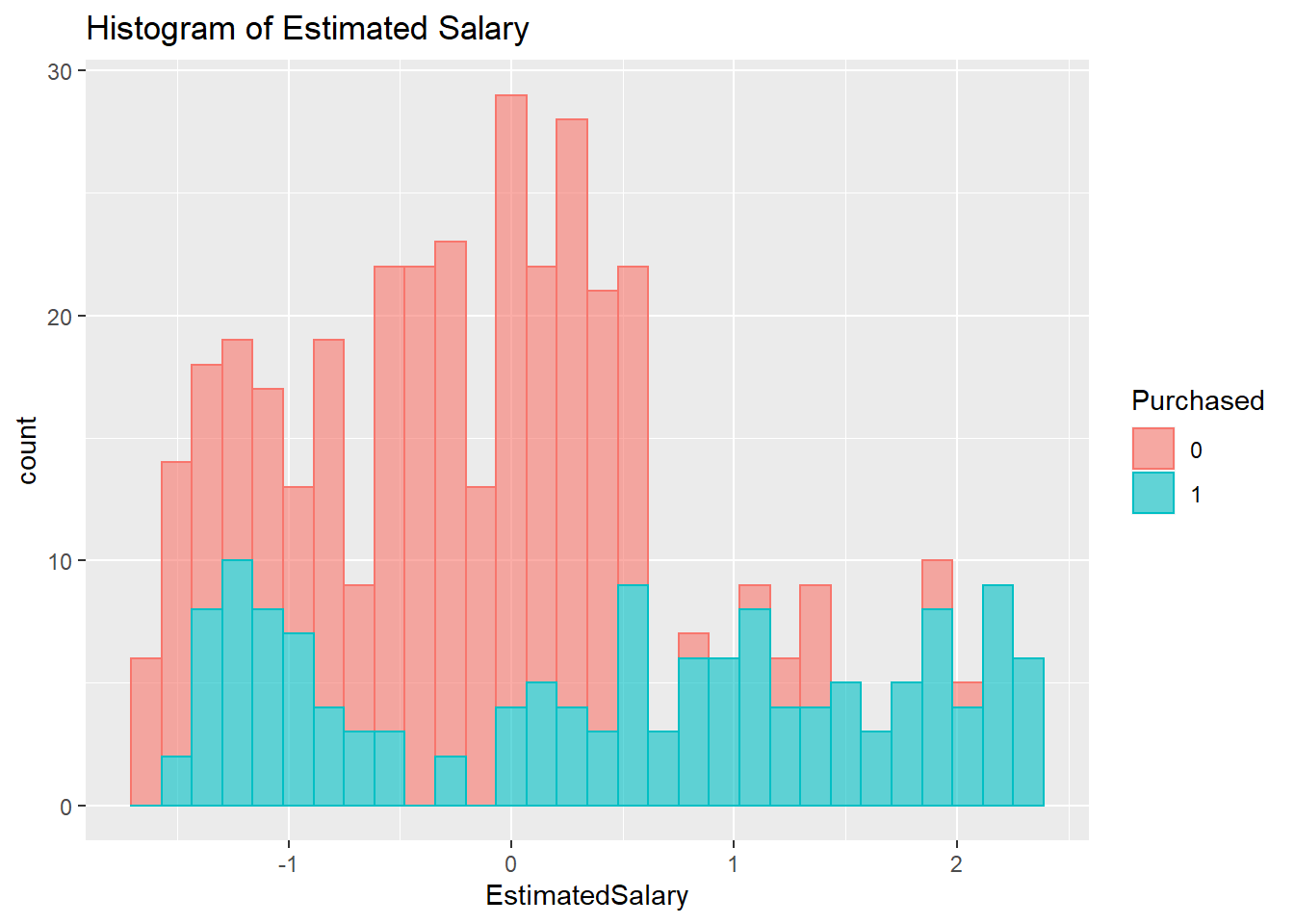``options(warn = oldw)``

# Grubbs’s Outlier Test

## Explanation

This is a test to check for the existence of outliers associated with each independent variable in the data frame. This test is based on Z-Scores. The function’s null hypothesis is that there are no outliers. If the p-value is smaller than 0.05, then the null hypothesis could be rejected, and the alternative hypothesis that there is at least one outlier could be accepted. The two-tail test is carried out for this data frame.

## Observation

All variables have p-values smaller than 0.05. Given a significant cut-off point of 0.05, all these variables have outliers.

``````# Detect outliers via z-score
grubbs.test(input_data\$Age,two.sided=TRUE,type=11)``````
``````##
##  Grubbs test for two opposite outliers
##
## data:  input_data\$Age
## G = 4.0065, U = 0.9798, p-value < 2.2e-16
## alternative hypothesis: -1.87496245115082 and 2.1315714052895 are outliers``````
``grubbs.test(input_data\$EstimatedSalary,two.sided=TRUE,type=11)``
``````##
##  Grubbs test for two opposite outliers
##
## data:  input_data\$EstimatedSalary
## G = 3.95930, U = 0.97965, p-value < 2.2e-16
## alternative hypothesis: -1.60549502203623 and 2.35380219630219 are outliers``````

# Correlation Analysis

## Explanation

The correlation statistics reveal the degree of associations between variables in the data set. Given a range between 0 and 1, a correlation value less than 0.5 in either direction indicates a weak correlation, and a value equal to or greater than 0.5 in either direction indicates a moderate to strong correlation.

## Observation

It appears that none of the variables have correlation coefficient greater than 0.5.

# Correlation Plot

``````oldw <- getOption("warn")
options(warn = -1)
pairs.panels(input_data[,num.names],gap=0,bg=c("green","red","yellow","blue","pink","purple"),pch= 21, cex=0.5)``````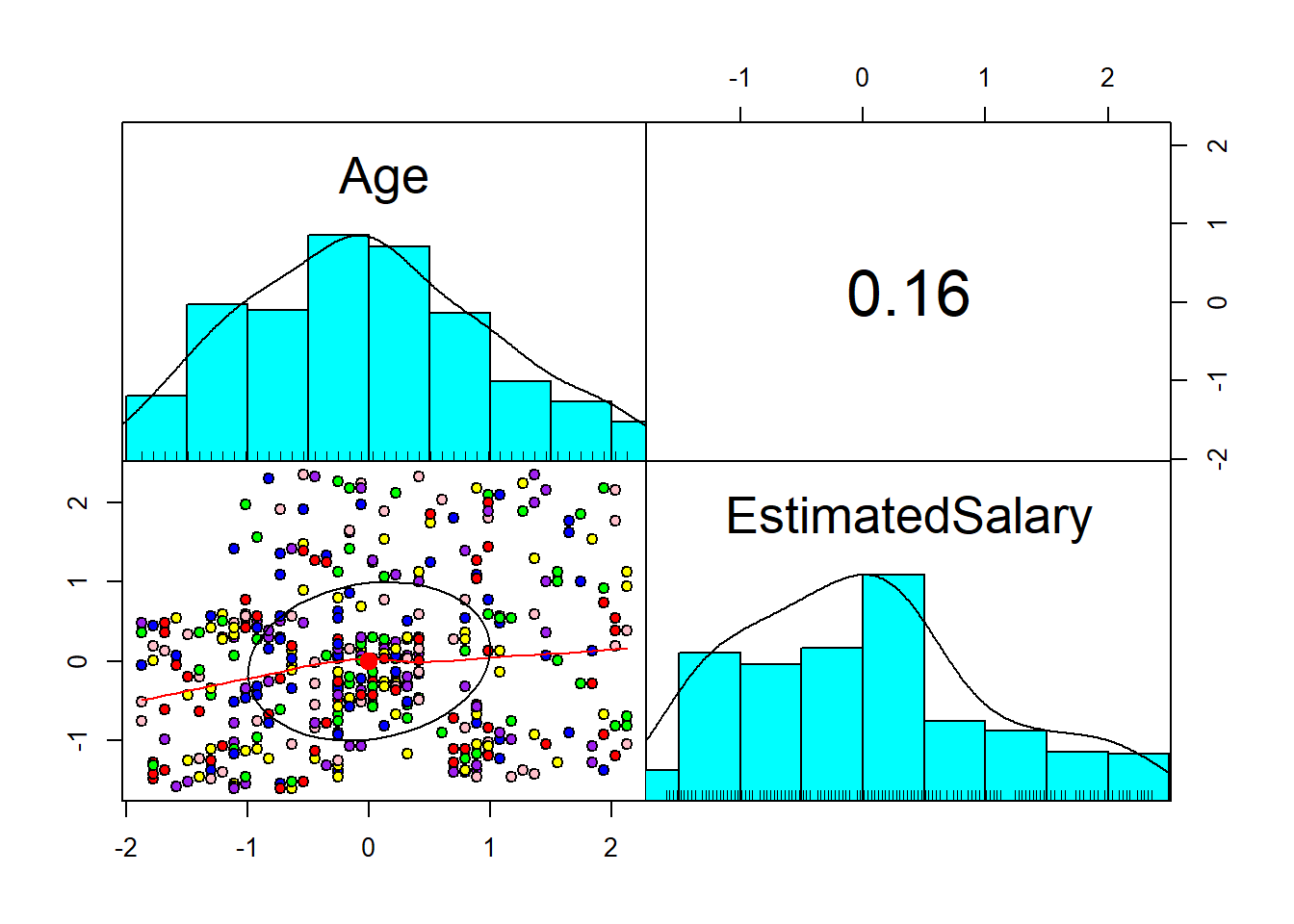``options(warn = oldw)``

# Correlation Table

``````oldw <- getOption("warn")
options(warn = -1)
cor(input_data[,num.names])``````
``````##                      Age EstimatedSalary
## Age             1.000000        0.155238
## EstimatedSalary 0.155238        1.000000``````
``options(warn = oldw)``

# Preparation of Training and Test Data Sets

## Explanation

The purpose of creating separate data sets for training and testing the model is because we want to see how differently the model would perform with data that it has never seen before.

``````oldw <- getOption("warn")
options(warn = -1)

set.seed(123)
ind <- sample(2, nrow(input_data), replace=T, prob=c(0.6,0.4))
df_sample_train <- input_data[ind==1,]
df_sample_test <- input_data[ind==2,]

options(warn = oldw)``````

# Comparison Between Kernelized SVMs and Other Classifiers

## Observation

As one could see from the Table of Model Predictive Statistics below, the Radial SVM model can make predictions with much higher accuracy than the Linear SVM model. The Radial SVM model has better Kappa statistics, higher sensitivity percentage, and higher specificity percentages. When comparing with other classifiers, the Radial SVM model also has a much stronger predictive ability. Even though Naive Bayes has an equivalent specificity percentage, its other model statistics are much smaller than that of the Radial SVM model’s.

## Table of Model Predictive Statistics

``````oldw <- getOption("warn")
options(warn = -1)
DF <- data.frame(
Classifier=c("Radial SVM", "Linear SVM", "Random Forest", "Naive Bayes"),
Accuracy=c(0.891,0.8205,0.8526,0.8654),
Kappa_Statistic=c(0.7735,0.6164,0.6886,0.7157),
Sensitivity=c(0.9636,0.8000,0.8727,0.8909),
Specificity=c(0.8515,0.8317,0.8416,0.8515)
)
knitr::kable(DF)``````
Classifier Accuracy Kappa_Statistic Sensitivity Specificity
Radial SVM 0.8910 0.7735 0.9636 0.8515
Linear SVM 0.8205 0.6164 0.8000 0.8317
Random Forest 0.8526 0.6886 0.8727 0.8416
Naive Bayes 0.8654 0.7157 0.8909 0.8515
``options(warn = oldw)``

# Kernelized SVMs Without Cross-Validation

## Model Statistics

``````oldw <- getOption("warn")
options(warn = -1)
set.seed(123)
SVMRadial = svm(formula = Purchased ~ Age+EstimatedSalary,
data = df_sample_train,
type = 'C-classification',

SVMLinear = svm(formula = Purchased ~ Age+EstimatedSalary,
data = df_sample_train,
type = 'C-classification',
kernel = 'linear')

SVMPredLinear = predict(SVMLinear, newdata = df_sample_test)

# Testing Result Confusion Matrix
``````## Confusion Matrix and Statistics
##
##
##             0 87  3
##             1 14 52
##
##                Accuracy : 0.891
##                  95% CI : (0.8313, 0.9352)
##     No Information Rate : 0.6474
##     P-Value [Acc > NIR] : 3.207e-12
##
##                   Kappa : 0.7717
##
##  Mcnemar's Test P-Value : 0.01529
##
##             Sensitivity : 0.9455
##             Specificity : 0.8614
##          Pos Pred Value : 0.7879
##          Neg Pred Value : 0.9667
##              Prevalence : 0.3526
##          Detection Rate : 0.3333
##    Detection Prevalence : 0.4231
##       Balanced Accuracy : 0.9034
##
##        'Positive' Class : 1
## ``````
``confusionMatrix(table(SVMPredLinear,df_sample_test[,5]), positive='1')``
``````## Confusion Matrix and Statistics
##
##
## SVMPredLinear  0  1
##             0 84 10
##             1 17 45
##
##                Accuracy : 0.8269
##                  95% CI : (0.7583, 0.8827)
##     No Information Rate : 0.6474
##     P-Value [Acc > NIR] : 5.773e-07
##
##                   Kappa : 0.6316
##
##  Mcnemar's Test P-Value : 0.2482
##
##             Sensitivity : 0.8182
##             Specificity : 0.8317
##          Pos Pred Value : 0.7258
##          Neg Pred Value : 0.8936
##              Prevalence : 0.3526
##          Detection Rate : 0.2885
##    Detection Prevalence : 0.3974
##       Balanced Accuracy : 0.8249
##
##        'Positive' Class : 1
## ``````
``options(warn = oldw)``

# Kernelized SVMs Without Cross-Validation

## Plots of Training Results

``````oldw <- getOption("warn")
options(warn = -1)
set.seed(123)
# Calculate the grid dimensions
X = seq(min(df_sample_train[,3])-2, max(df_sample_train[,3])+2, by = 0.006)
Y = seq(min(df_sample_train[,4])-2, max(df_sample_train[,4])+2, by = 0.006)
grid_set = expand.grid(X, Y)

# Replace X and Y with actual names
colnames(grid_set) = c('Age', 'EstimatedSalary')

# Calculate the outlines for the two groups of data points

# Plot SVM Radial Kernel result
plot(df_sample_train[,c(3,4)],
main = 'SVM Radial Kernel (Training Data)',
xlab = 'Age',
ylab = 'Estimated Salary',
xlim = range(X),
ylim = range(Y))

#Create outlines for the two groups of data points

#Apply color to the two areas specificed by the contour function above
points(grid_set, pch = '.', col = ifelse(SVMGridRadial == 1, '#339CFF', '#FF8333'), cex = 1)

#Add the data points onto the plot
points(df_sample_train[,c(3,4,5)], pch = 21, bg = ifelse(df_sample_train[,5] == 1, '#3C33FF','#FF3333'), cex = 1)``````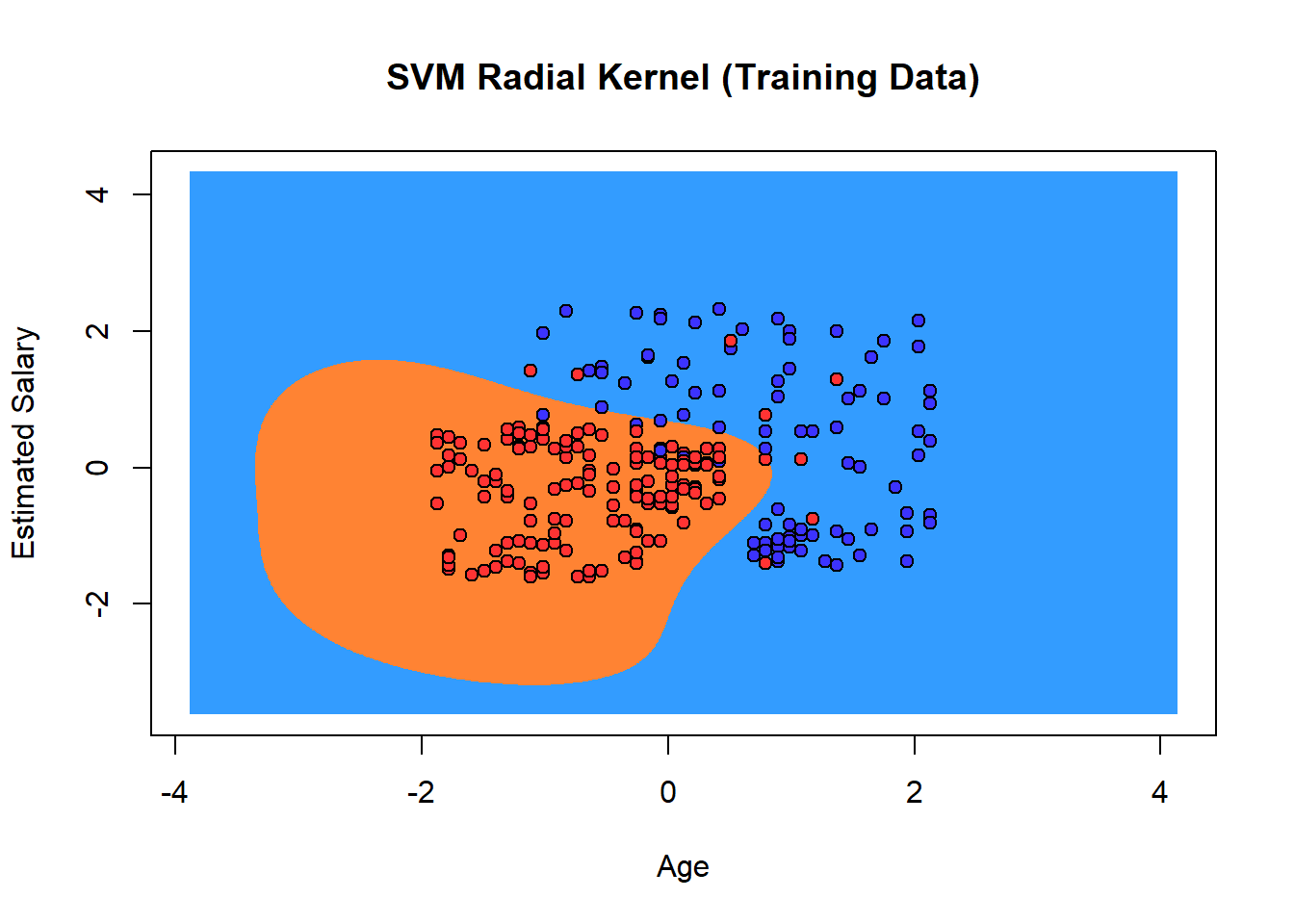``````# Plot SVM Linear Kernel result
plot(df_sample_train[,c(3,4)],
main = 'SVM Linear Kernel  (Training Data)',
xlab = 'Age',
ylab = 'Estimated Salary',
xlim = range(X),
ylim = range(Y))

# Calculate the outlines for the two groups of data points
SVMGridLinear = predict(SVMLinear, newdata = grid_set)

#Create outlines for the two groups of data points
contour(X, Y, matrix(as.numeric(SVMGridLinear), length(X), length(Y)), add = TRUE)

#Apply color to the two areas specificed by the contour function above
points(grid_set, pch = '.', col = ifelse(SVMGridLinear == 1, '#339CFF', '#FF8333'), cex = 1)

#Add the data points onto the plot
points(df_sample_train[,c(3,4,5)], pch = 21, bg = ifelse(df_sample_train[,5] == 1, '#3C33FF','#FF3333'), cex = 1)``````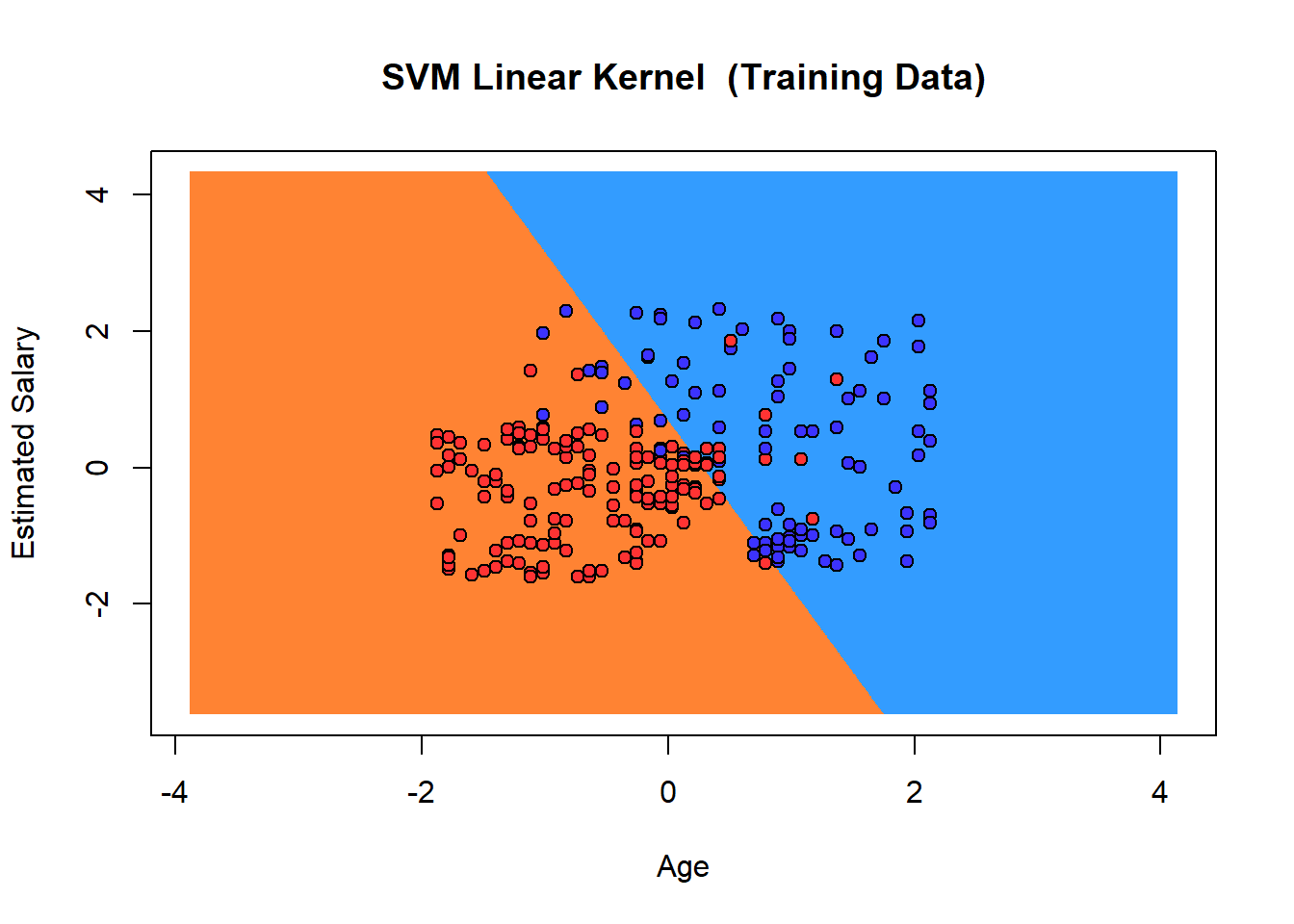``options(warn = oldw)``

# Kernelized SVMs Without Cross-Validation

## Plots of Testing Results

``````oldw <- getOption("warn")
options(warn = -1)
set.seed(123)

# Calculate the grid dimensions
X = seq(min(df_sample_test[,3])-2, max(df_sample_test[,3])+2, by = 0.006)
Y = seq(min(df_sample_test[,4])-2, max(df_sample_test[,4])+2, by = 0.006)
grid_set = expand.grid(X, Y)

# Replace X and Y with actual names
colnames(grid_set) = c('Age', 'EstimatedSalary')

# Calculate the outlines for the two groups of data points

# Plot SVM Radial Kernel result
plot(df_sample_test[,c(3,4)],
main = 'SVM Radial Kernel (Testing Data)',
xlab = 'Age',
ylab = 'Estimated Salary',
xlim = range(X),
ylim = range(Y))

#Create outlines for the two groups of data points

#Apply color to the two areas specificed by the contour function above
points(grid_set, pch = '.', col = ifelse(SVMGridRadial == 1, '#339CFF', '#FF8333'), cex = 1)

#Add the data points onto the plot
points(df_sample_test[,c(3,4,5)], pch = 21, bg = ifelse(df_sample_test[,5] == 1, '#3C33FF','#FF3333'), cex = 1)``````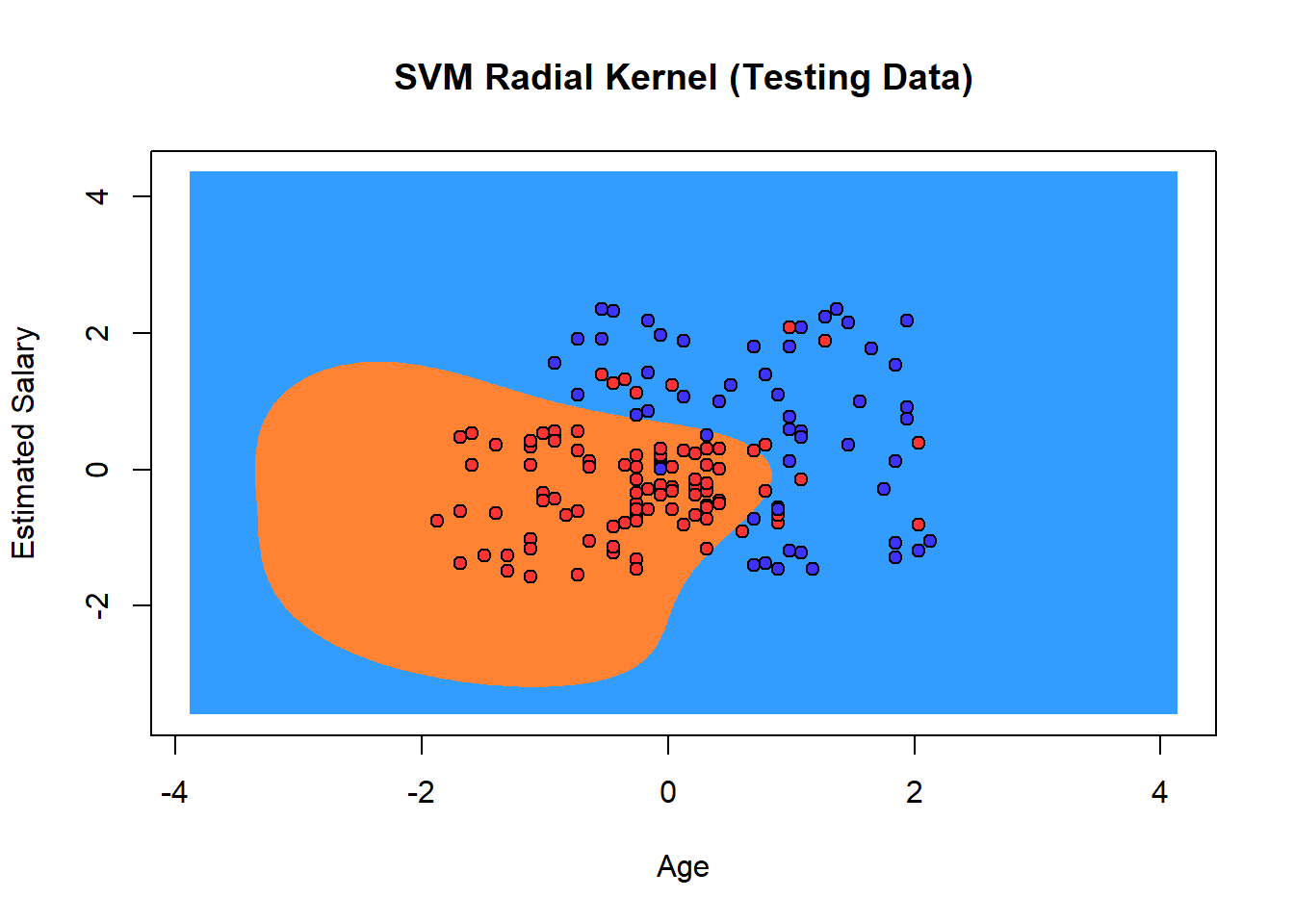``````# Plot SVM Linear Kernel result
plot(df_sample_test[,c(3,4)],
main = 'SVM Linear Kernel  (Testing Data)',
xlab = 'Age',
ylab = 'Estimated Salary',
xlim = range(X),
ylim = range(Y))

# Calculate the outlines for the two groups of data points
SVMGridLinear = predict(SVMLinear, newdata = grid_set)

#Create outlines for the two groups of data points
contour(X, Y, matrix(as.numeric(SVMGridLinear), length(X), length(Y)), add = TRUE)

#Apply color to the two areas specificed by the contour function above
points(grid_set, pch = '.', col = ifelse(SVMGridLinear == 1, '#339CFF', '#FF8333'), cex = 1)

#Add the data points onto the plot
points(df_sample_test[,c(3,4,5)], pch = 21, bg = ifelse(df_sample_test[,5] == 1, '#3C33FF','#FF3333'), cex = 1)``````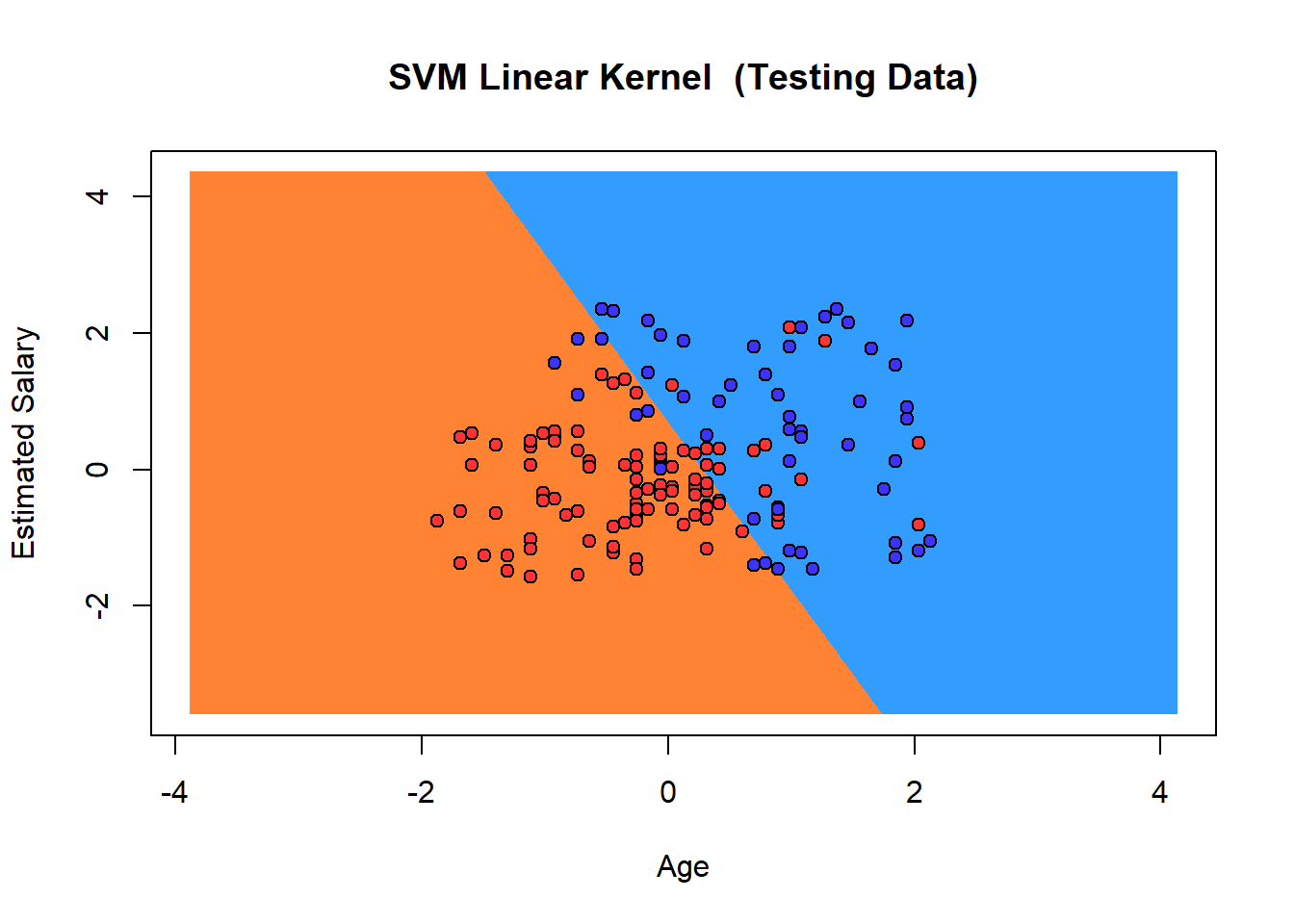``options(warn = oldw)``

# Kernelized SVMs With Cross-Validation

``````oldw <- getOption("warn")
options(warn = -1)
set.seed(123)

gridRadial = expand.grid(sigma = 2^c(-25, -20, -15,-10, -5, 0), C = 2^c(0:5))

numCores <- detectCores()
c1 <- makeCluster(numCores,type="SOCK")
registerDoSNOW(c1)
Purchased ~ Age+EstimatedSalary,
data=df_sample_train[,c(3,4,5)],
tuneLength=20,
savePredictions=T,
trControl = trainControl(method = "repeatedcv", number = 10, repeats = 10, verboseIter = F, savePredictions=T),
metric="Accuracy"
)
stopCluster(c1)
``````## Support Vector Machines with Radial Basis Function Kernel
##
## 244 samples
##   2 predictor
##   2 classes: '0', '1'
##
## No pre-processing
## Resampling: Cross-Validated (10 fold, repeated 10 times)
## Summary of sample sizes: 219, 219, 220, 221, 219, 220, ...
## Resampling results across tuning parameters:
##
##   sigma         C   Accuracy   Kappa
##   2.980232e-08   1  0.6393739  0.00000000
##   2.980232e-08   2  0.6393739  0.00000000
##   2.980232e-08   4  0.6393739  0.00000000
##   2.980232e-08   8  0.6393739  0.00000000
##   2.980232e-08  16  0.6393739  0.00000000
##   2.980232e-08  32  0.6393739  0.00000000
##   9.536743e-07   1  0.6393739  0.00000000
##   9.536743e-07   2  0.6393739  0.00000000
##   9.536743e-07   4  0.6393739  0.00000000
##   9.536743e-07   8  0.6393739  0.00000000
##   9.536743e-07  16  0.6393739  0.00000000
##   9.536743e-07  32  0.6393739  0.00000000
##   3.051758e-05   1  0.6393739  0.00000000
##   3.051758e-05   2  0.6393739  0.00000000
##   3.051758e-05   4  0.6393739  0.00000000
##   3.051758e-05   8  0.6393739  0.00000000
##   3.051758e-05  16  0.6393739  0.00000000
##   3.051758e-05  32  0.6393739  0.00000000
##   9.765625e-04   1  0.6393739  0.00000000
##   9.765625e-04   2  0.6479768  0.02987053
##   9.765625e-04   4  0.7747536  0.44273132
##   9.765625e-04   8  0.8129471  0.55301139
##   9.765625e-04  16  0.8403391  0.62643561
##   9.765625e-04  32  0.8509225  0.65511706
##   3.125000e-02   1  0.8936355  0.76017681
##   3.125000e-02   2  0.9089051  0.79675351
##   3.125000e-02   4  0.9145370  0.81129066
##   3.125000e-02   8  0.9158884  0.81591191
##   3.125000e-02  16  0.9183384  0.82171018
##   3.125000e-02  32  0.9187384  0.82247359
##   1.000000e+00   1  0.9326051  0.85452171
##   1.000000e+00   2  0.9313370  0.85179435
##   1.000000e+00   4  0.9284355  0.84579897
##   1.000000e+00   8  0.9271493  0.84240222
##   1.000000e+00  16  0.9246993  0.83677319
##   1.000000e+00  32  0.9214659  0.82892744
##
## Accuracy was used to select the optimal model using the largest value.
## The final values used for the model were sigma = 1 and C = 1.``````
``plot(SVMRadial)``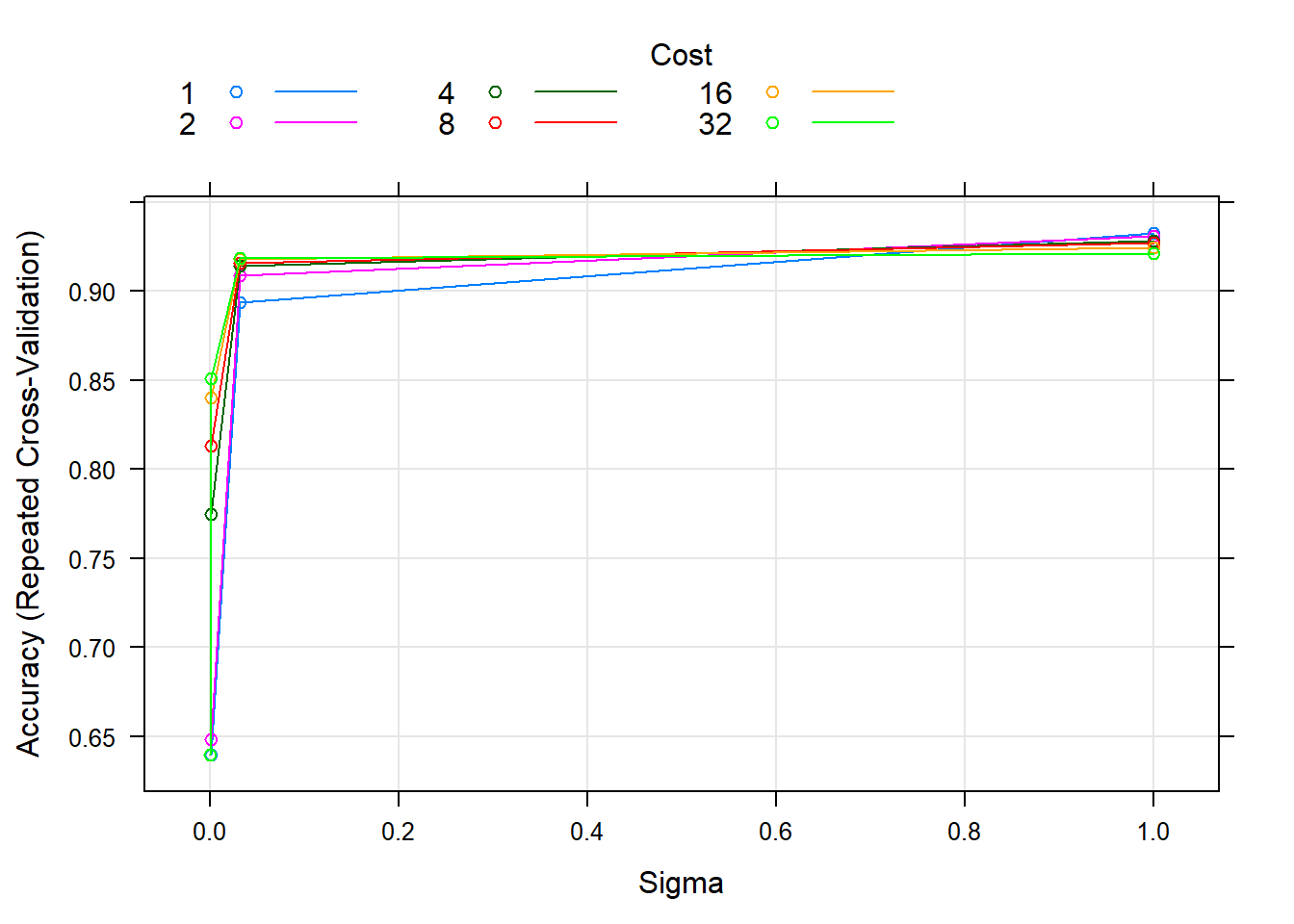``````# Training Result Confusion Matrix
``````## Confusion Matrix and Statistics
##
##          Actual
## Predicted     0     1
##         0 54890 20429
##         1  1270 11251
##
##                Accuracy : 0.753
##                  95% CI : (0.7501, 0.7558)
##     No Information Rate : 0.6393
##     P-Value [Acc > NIR] : < 2.2e-16
##
##                   Kappa : 0.383
##
##  Mcnemar's Test P-Value : < 2.2e-16
##
##             Sensitivity : 0.3551
##             Specificity : 0.9774
##          Pos Pred Value : 0.8986
##          Neg Pred Value : 0.7288
##              Prevalence : 0.3607
##          Detection Rate : 0.1281
##    Detection Prevalence : 0.1425
##       Balanced Accuracy : 0.6663
##
##        'Positive' Class : 1
## ``````
``````SVMPredRadial = predict(SVMRadial, newdata = df_sample_test[,c(3,4,5)])
``````##    1 1 0 0 1 0 1 0 0 0 0 0 1 0 1 0 0 0 1 0 1 1 1 1 0 1 1 0 1 0 0 1 1 0 1 0 1
##   1 1 0 1 0 1 0 1 0 1 1 0 1 1 1 1 0 0 0 0 0 1 1 0 1 0 0 0 1 1 0 1 0 1 0 0 1
##   0 0 0 1 0 1 0 1 0 1 1 0 1 1 0 0 0 0 0 0 1 0 0 1 0 1 0 0 1 1 0 0 1 1 0 0 0
##  0 1 0 1 0 0 0 1 1 0 0 0 0 0 1 1 0 1 1 0 0 1 0 1 0 0 1 0 0 1 0 0 0 0 1 0 0
##  0 0 1 1 1 0 0 1
## Levels: 0 1``````
``confusionMatrix (table(SVMPredRadial,df_sample_test[,5]), positive='1')``
``````## Confusion Matrix and Statistics
##
##
##             0 86  2
##             1 15 53
##
##                Accuracy : 0.891
##                  95% CI : (0.8313, 0.9352)
##     No Information Rate : 0.6474
##     P-Value [Acc > NIR] : 3.207e-12
##
##                   Kappa : 0.7735
##
##  Mcnemar's Test P-Value : 0.003609
##
##             Sensitivity : 0.9636
##             Specificity : 0.8515
##          Pos Pred Value : 0.7794
##          Neg Pred Value : 0.9773
##              Prevalence : 0.3526
##          Detection Rate : 0.3397
##    Detection Prevalence : 0.4359
##       Balanced Accuracy : 0.9076
##
##        'Positive' Class : 1
## ``````
``````gridLinear = expand.grid(C = 2^c(0:5))

numCores <- detectCores()
c1 <- makeCluster(numCores,type="SOCK")
registerDoSNOW(c1)
SVMLinear <- train(
Purchased ~ Age+EstimatedSalary,
data=df_sample_train[,c(3,4,5)],
method="svmLinear",
tuneGrid = gridLinear,
tuneLength=20,
savePredictions=T,
trControl = trainControl(method = "repeatedcv", number = 10, repeats = 10, verboseIter = F, savePredictions=T),
metric="Accuracy"
)
stopCluster(c1)
SVMLinear``````
``````## Support Vector Machines with Linear Kernel
##
## 244 samples
##   2 predictor
##   2 classes: '0', '1'
##
## No pre-processing
## Resampling: Cross-Validated (10 fold, repeated 10 times)
## Summary of sample sizes: 220, 219, 220, 219, 219, 220, ...
## Resampling results across tuning parameters:
##
##   C   Accuracy   Kappa
##    1  0.8623725  0.6991453
##    2  0.8647710  0.7054676
##    4  0.8623377  0.7000224
##    8  0.8590877  0.6926416
##   16  0.8590710  0.6918673
##   32  0.8582362  0.6897540
##
## Accuracy was used to select the optimal model using the largest value.
## The final value used for the model was C = 2.``````
``plot(SVMLinear)``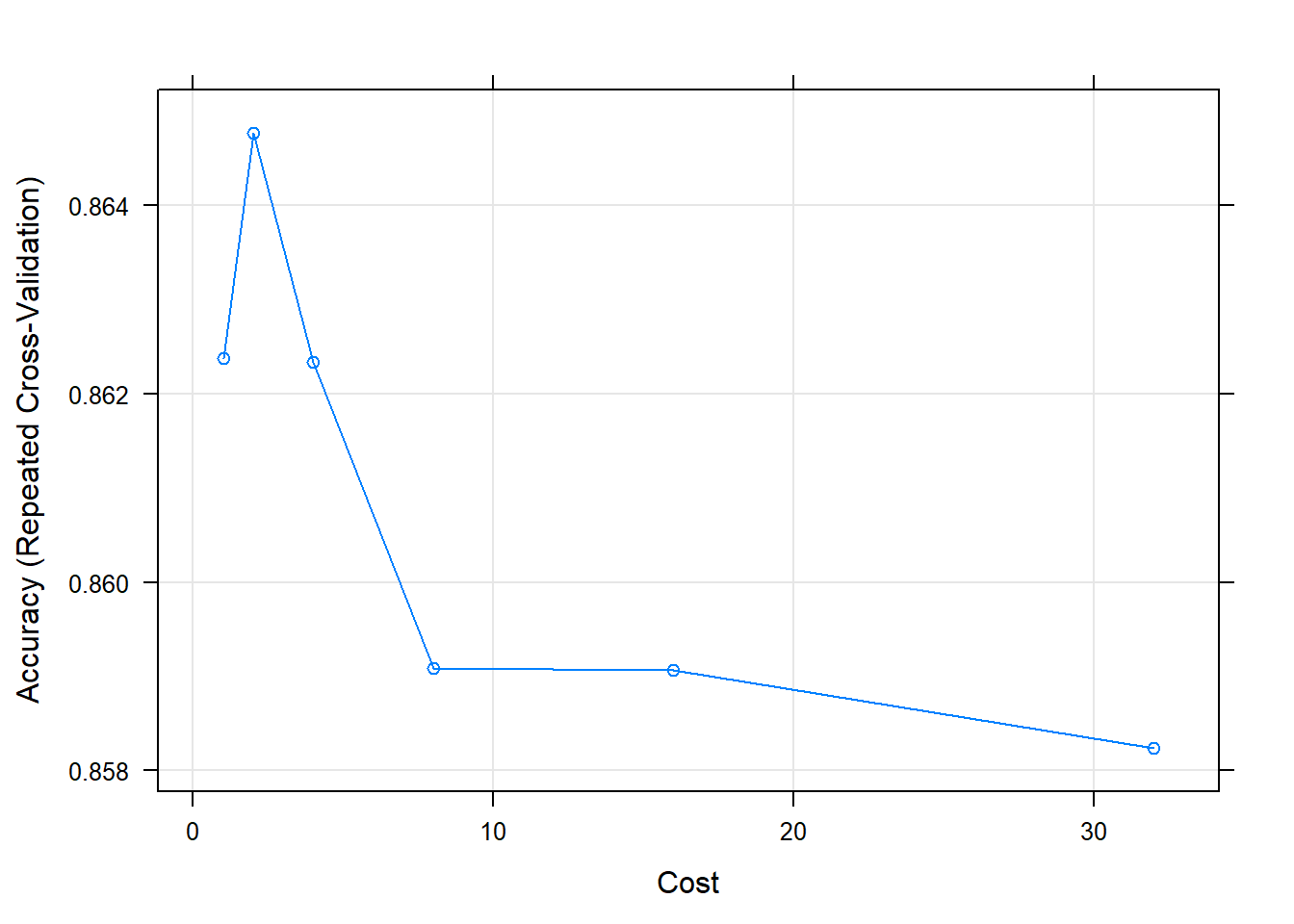``````# Training Result Confusion Matrix
confusionMatrix( table( Predicted=(SVMLinear\$pred)\$pred,Actual=(SVMLinear\$pred)\$obs ), positive="1" )``````
``````## Confusion Matrix and Statistics
##
##          Actual
## Predicted    0    1
##         0 8395 1071
##         1  965 4209
##
##                Accuracy : 0.8609
##                  95% CI : (0.8552, 0.8665)
##     No Information Rate : 0.6393
##     P-Value [Acc > NIR] : < 2e-16
##
##                   Kappa : 0.6971
##
##  Mcnemar's Test P-Value : 0.01996
##
##             Sensitivity : 0.7972
##             Specificity : 0.8969
##          Pos Pred Value : 0.8135
##          Neg Pred Value : 0.8869
##              Prevalence : 0.3607
##          Detection Rate : 0.2875
##    Detection Prevalence : 0.3534
##       Balanced Accuracy : 0.8470
##
##        'Positive' Class : 1
## ``````
``````SVMPredLinear = predict(SVMLinear, newdata = df_sample_test[,c(3,4,5)])
SVMPredLinear``````
``````##    1 0 0 0 1 0 1 0 0 0 0 1 0 0 1 1 0 0 1 0 1 0 0 1 0 1 1 0 1 1 0 1 1 0 1 0 1
##   1 1 0 1 0 0 0 1 0 1 1 0 0 1 1 1 0 0 0 0 0 1 1 0 1 0 0 0 1 1 0 1 0 1 0 0 0
##   0 0 0 1 0 1 0 0 0 0 1 0 1 1 0 0 0 0 0 1 1 1 0 1 0 1 0 0 1 0 0 0 1 1 0 0 1
##  0 1 0 1 0 0 0 0 1 0 0 0 0 0 1 1 0 1 0 0 0 1 0 1 0 0 0 0 0 0 1 1 0 0 1 0 0
##  0 0 1 1 0 0 0 1
## Levels: 0 1``````
``confusionMatrix (table(SVMPredLinear,df_sample_test[,5]), positive='1')``
``````## Confusion Matrix and Statistics
##
##
## SVMPredLinear  0  1
##             0 84 11
##             1 17 44
##
##                Accuracy : 0.8205
##                  95% CI : (0.7511, 0.8773)
##     No Information Rate : 0.6474
##     P-Value [Acc > NIR] : 1.487e-06
##
##                   Kappa : 0.6164
##
##  Mcnemar's Test P-Value : 0.3447
##
##             Sensitivity : 0.8000
##             Specificity : 0.8317
##          Pos Pred Value : 0.7213
##          Neg Pred Value : 0.8842
##              Prevalence : 0.3526
##          Detection Rate : 0.2821
##    Detection Prevalence : 0.3910
##       Balanced Accuracy : 0.8158
##
##        'Positive' Class : 1
## ``````
``options(warn = oldw)``

# Random Forest

## Training the model without cross-validation

``````oldw <- getOption("warn")
options(warn = -1)
set.seed(123)
rf_train <- randomForest(Purchased ~ Age+EstimatedSalary,
data=df_sample_train, importance=TRUE,
ntree = 100
)
varImp(rf_train)``````
``````##                        0        1
## Age             35.02138 35.02138
## EstimatedSalary 20.92961 20.92961``````
``varImpPlot(rf_train)``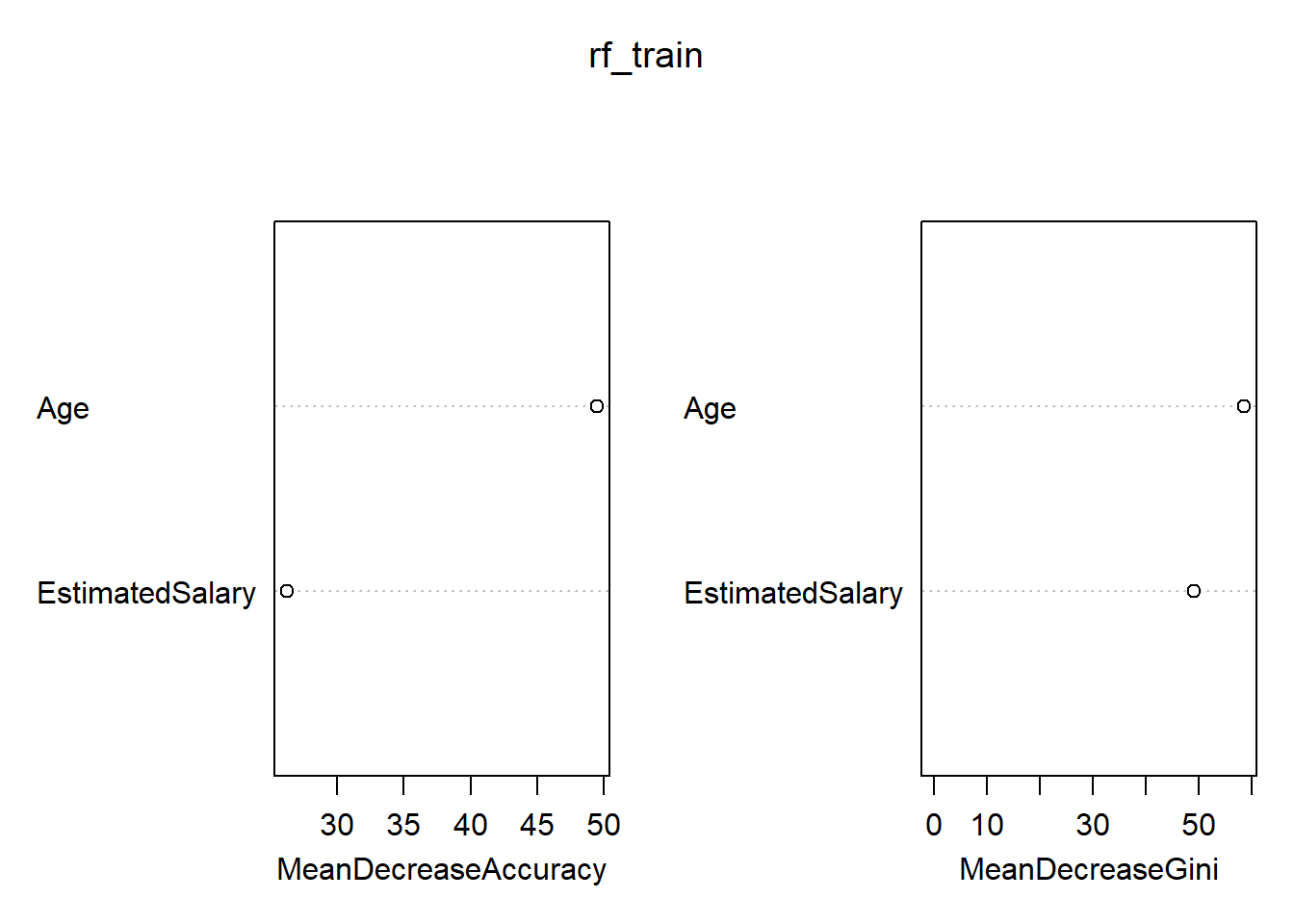``````rf_pred <- predict(rf_train, newdata=df_sample_test)
confusionMatrix( table(Predicted=rf_pred,Actual=df_sample_test\$Purchased), positive="1" )``````
``````## Confusion Matrix and Statistics
##
##          Actual
## Predicted  0  1
##         0 87  6
##         1 14 49
##
##                Accuracy : 0.8718
##                  95% CI : (0.809, 0.9199)
##     No Information Rate : 0.6474
##     P-Value [Acc > NIR] : 2.108e-10
##
##                   Kappa : 0.7282
##
##  Mcnemar's Test P-Value : 0.1175
##
##             Sensitivity : 0.8909
##             Specificity : 0.8614
##          Pos Pred Value : 0.7778
##          Neg Pred Value : 0.9355
##              Prevalence : 0.3526
##          Detection Rate : 0.3141
##    Detection Prevalence : 0.4038
##       Balanced Accuracy : 0.8761
##
##        'Positive' Class : 1
## ``````
``options(warn = oldw)``

# Random Forest

## Training the model using cross-validation

``````oldw <- getOption("warn")
options(warn = -1)

# Create a stratified index of folds
set.seed(123)

# Set up doSNOW package for multi-core training. This will speed up the training process.
numCores <- detectCores()
c1 <- makeCluster(numCores,type="SOCK")
registerDoSNOW(c1)

# Train and test the model with the number of folds
set.seed(123)
train_obj <- train(
Purchased ~ Age + EstimatedSalary,
data=df_sample_train,
method="rf",
tuneLength = 20,
ntree=100,
trControl = trainControl(method = "repeatedcv", number = 10, repeats = 10, verboseIter = F, savePredictions=T),
metric="Accuracy"
)``````
``## note: only 1 unique complexity parameters in default grid. Truncating the grid to 1 .``
``````# Shutdown cluster
stopCluster(c1)
train_obj``````
``````## Random Forest
##
## 244 samples
##   2 predictor
##   2 classes: '0', '1'
##
## No pre-processing
## Resampling: Cross-Validated (10 fold, repeated 10 times)
## Summary of sample sizes: 219, 219, 220, 221, 219, 220, ...
## Resampling results:
##
##   Accuracy   Kappa
##   0.9023297  0.7875665
##
## Tuning parameter 'mtry' was held constant at a value of 2``````
``````# varImp(train_obj)
# summary(train_obj)
# plot(train_obj)

table((train_obj\$pred)\$pred)``````
``````##
##    0    1
## 1559  881``````
``table((train_obj\$pred)\$obs)``
``````##
##    0    1
## 1560  880``````
``````# Confusion Matrix: Comparison between the predicted values and actual observed values. Calculate the average accuracy statistics.
confusionMatrix( table( Predicted=(train_obj\$pred)\$pred,Actual=(train_obj\$pred)\$obs ), positive="1" )``````
``````## Confusion Matrix and Statistics
##
##          Actual
## Predicted    0    1
##         0 1440  119
##         1  120  761
##
##                Accuracy : 0.902
##                  95% CI : (0.8896, 0.9136)
##     No Information Rate : 0.6393
##     P-Value [Acc > NIR] : <2e-16
##
##                   Kappa : 0.7877
##
##  Mcnemar's Test P-Value : 1
##
##             Sensitivity : 0.8648
##             Specificity : 0.9231
##          Pos Pred Value : 0.8638
##          Neg Pred Value : 0.9237
##              Prevalence : 0.3607
##          Detection Rate : 0.3119
##    Detection Prevalence : 0.3611
##       Balanced Accuracy : 0.8939
##
``````